# NEET Important Topic - Physics Mathematical Tools

Get plus subscription and access unlimited live and recorded courses## Physics Mathematical Tools for NEET

As far as the NEET aspirants are concerned, one of the challenges they face while studying physics for the NEET exam is the mathematical operations and tools used at the time of solving numerical problems. However, this can be made easier if we learn the basic mathematical tools required in physics for the NEET exam.

In this article discussing the new and old mathematical tools in physics, we will see different physical constants and conversion coefficients that will come in handy while solving numerical problems. The importance of mathematics of tools like trigonometric functions of angles and their applications are discussed here. The application of differentiation and integration plays an important role in solving most of the physics problems in several chapters.

Now, let us move on to the important concepts and formulae of mathematical tools required in physics for the NEET exam, along with a few solved mathematical tools examples.

### Important Topics of Mathematical Tools

• Binomial expansion

• Important trigonometric identities.

• Differentiation

• Rules of differentiation

• Applications of differentiation

• Integration and its application

• Definite integral

• Integration Formulae

### Important Concepts of Mathematical Tools

 Name of the Concept Key Points of Concept 1. Quadratic equation A quadratic equation is a second-degree equation in which the highest power to which the variable is raised is to the power 2.The quadratic equation form is given below where a, b and c are constants$ax^2+bx+c=0$The discriminant of the quadratic equation is given by,$D=b^2-4ac$The solution of the quadratic equation is given by,$x=\dfrac{-b\pm\sqrt{b^2-4ac}}{2a}$One of the applications of quadratic equations in physics is calculating the time when the ball kicked by reaches at a height H. Here, we will get two values of time t1 and t1 after solving the quadratic equations.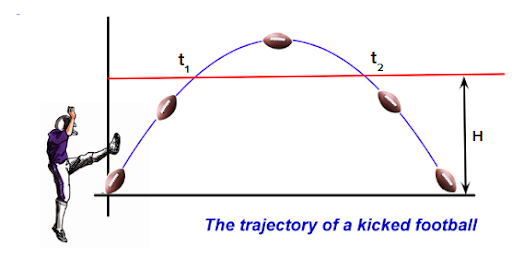2. Binomial expansion The pattern of binomial expansion is given by the binomial theorem as follows$(a+b)^n=a^n+na^{n-1}b^1+\dfrac{n(n-1)}{2\times1}a^{n-2}b^2+\dfrac{n(n-1)(n-2)}{3\times2\times1}a^{n-3}b^3...~...+b^n$Similarly, the expansion of (1+x)n is given by,$(1+x)^n=1+nx^1+\dfrac{n(n-1)}{2\times1}x^2+\dfrac{n(n-1)(n-2)}{3\times2\times1}x^3...~...+x^n$If the values x is very small, we can neglect the higher powers of x and rewrite the binomial expansion as$(1+x)^n=1+nx$ 3. Important trigonometric identities. Trigonometric ratios has positive or negative values depending on the quadrant a given below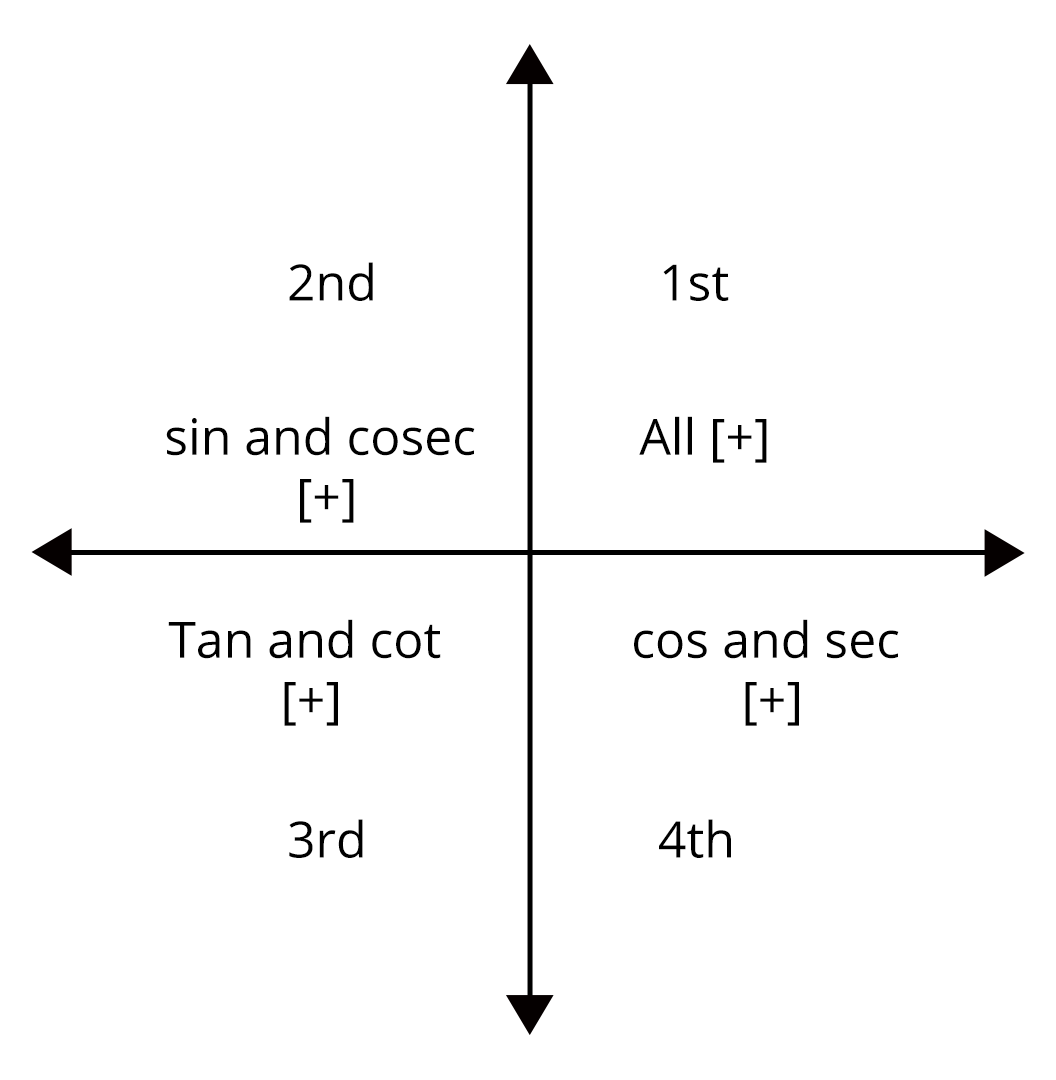Image: Trigonometric function signsSome of the important trigonometric relations are given below.$\sin^2\theta+\cos^2\theta=1$$1+\tan^2\theta=\sec^2\theta$$\sin(A+B)=\sin A\cos B+\cos A\sin B$$\sin(A-B)=\sin A\cos B-\cos A\sin B$$\cos(A+B)=\cos A\cos B-\sin A\sin B$$\cos(A-B)=\cos A\cos B+\sin A\sin B$$\tan(A+B)=\dfrac{\tan A+\tan B}{1-\tan A\tan B}$ 4. Differentiation Let y be a function of x, then ratio of change of y for a very small change in x can be represented by $\dfrac{dy}{dx}=\lim_{\Delta x \rightarrow 0}\dfrac{\Delta y}{\Delta x}$One of the application of differentiation is finding the slope of y-x graph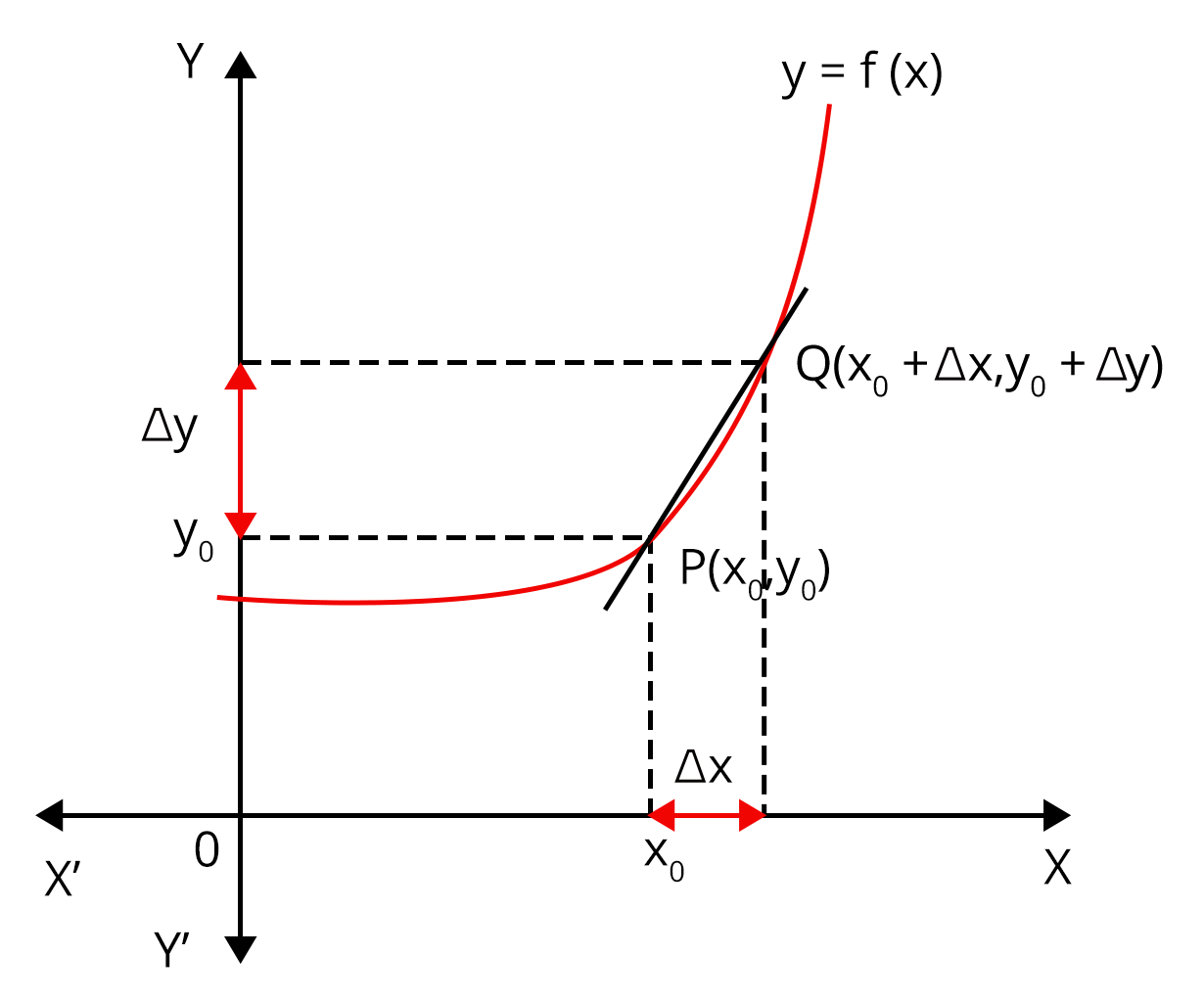Image: application of differentiation$\text{slope of PQ}=\dfrac{dy}{dx}$Some of the differentiation of basic function is given below.$\dfrac{d}{dx}(x^n)=nx^{n-1}$$\dfrac{d}{dx}(x)=1$$\dfrac{d}{dx}(\text{constant})=0$$\dfrac{d}{dx}(\ln x)=\dfrac{1}{x}$$\dfrac{d}{dx}(e^ x)=e^ x$$\dfrac{d}{dx}(a^ x)=a^ x\ln e$$\dfrac{d}{dx}(\sin x )=\cos x$$\dfrac{d}{dx}(\cos x )=-\sin x 5. Rules of differentiation Let f(x) and g(x) be two functions of x. The addition and subtraction rule of differentiation is given by\dfrac{d}{dx}(f(x)\pm g(x))=\dfrac{d}{dx}f(x)\pm \dfrac{d}{dx}g(x)Constant rule:\dfrac{d}{dx}(kf(x))=k\dfrac{d}{dx}f(x)Where k is a constantProduct rule: \dfrac{d}{dx}(f(x)\cdot g(x))=f(x)\cdot\dfrac{d}{dx}g(x)+g(x)\cdot\dfrac{d}{dx}f(x)Quotient rule:\dfrac{d}{dx}\left(\dfrac{f(x)}{g(x)}\right)=\dfrac{g(x)\cdot\dfrac{d}{dx}f(x)-f(x)\cdot\dfrac{d}{dx}g(x)}{\left(g(x)\right)^2} 6. Applications of differentiation Velocity is the rate of change of displacement with respect to timev=\dfrac{dx}{dt}The slope of the displacement time graph gives velocity.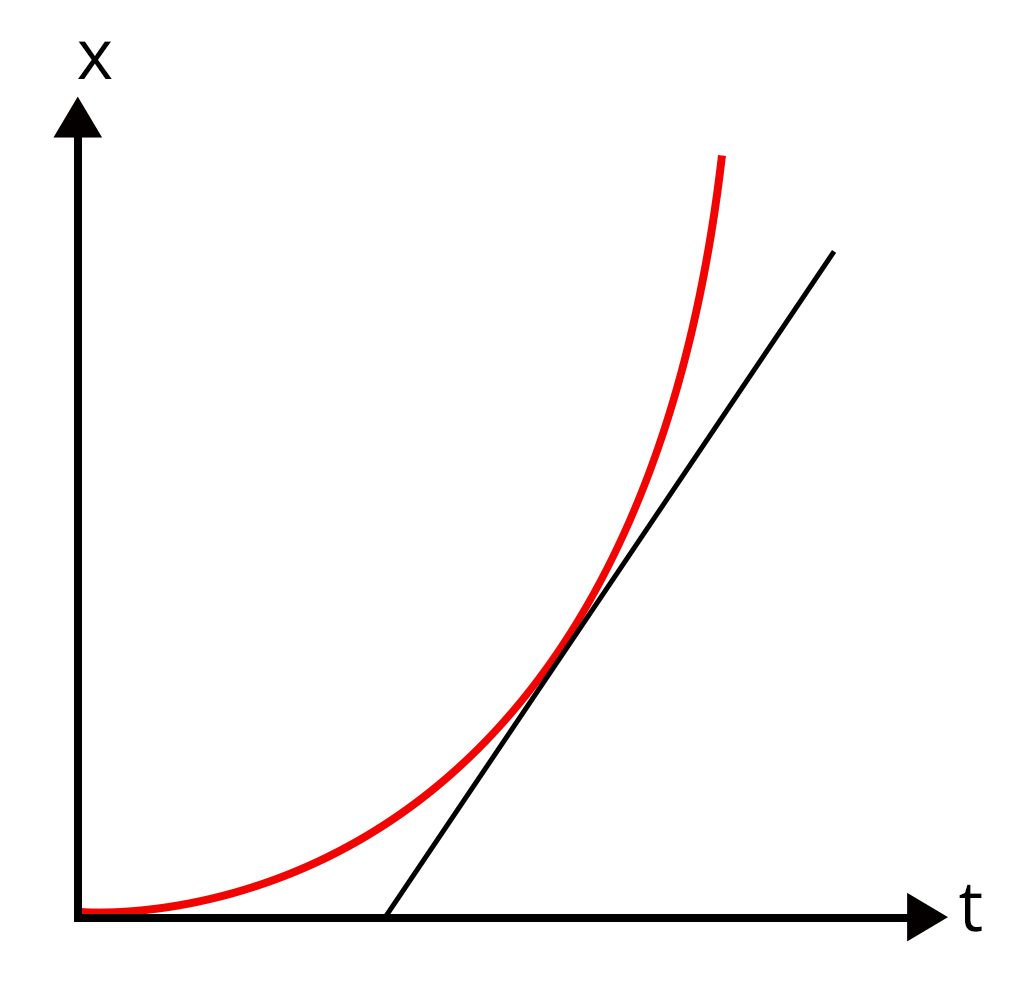Image: slope of displacement time graph Acceleration is the rate of change of velocity with respect to time.a=\dfrac{dv}{dt}$$a=\dfrac{d}{dt}\left(\dfrac{dx}{dt}\right )$$a=\dfrac{d^2x}{dt^2}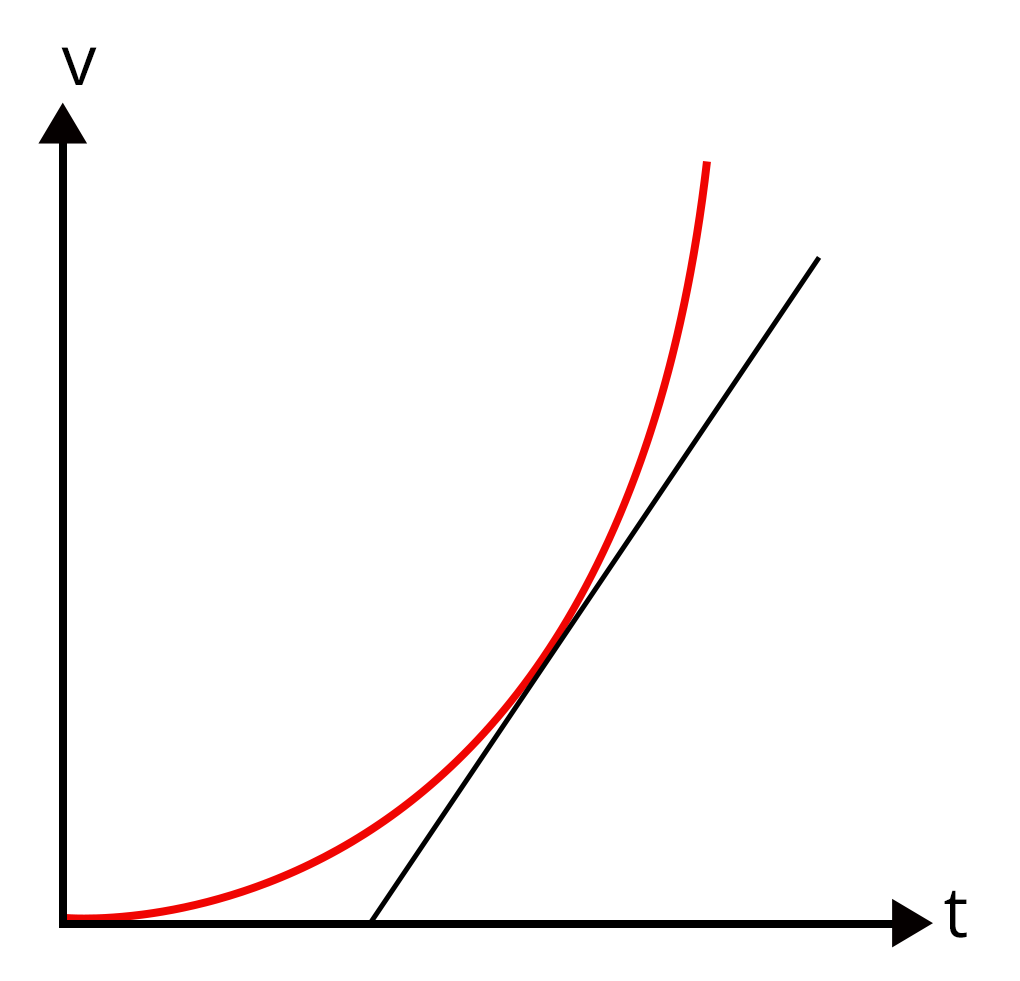Image: slope of velocity time graph The slope of the velocity time graph gives the acceleration. 7. Integration and its application Integration is basically the summation of infinite quantities, of infinitely small values.If f(x) is the derivative of g(x) with respect to x, then g(x) is the integral of f(x)f(x)=\dfrac{d}{dx}g(x)$$\int f(x)dx=g(x)+c$Where c is an arbitrary constant.We can calculate the area under the curve of a given graph using integration.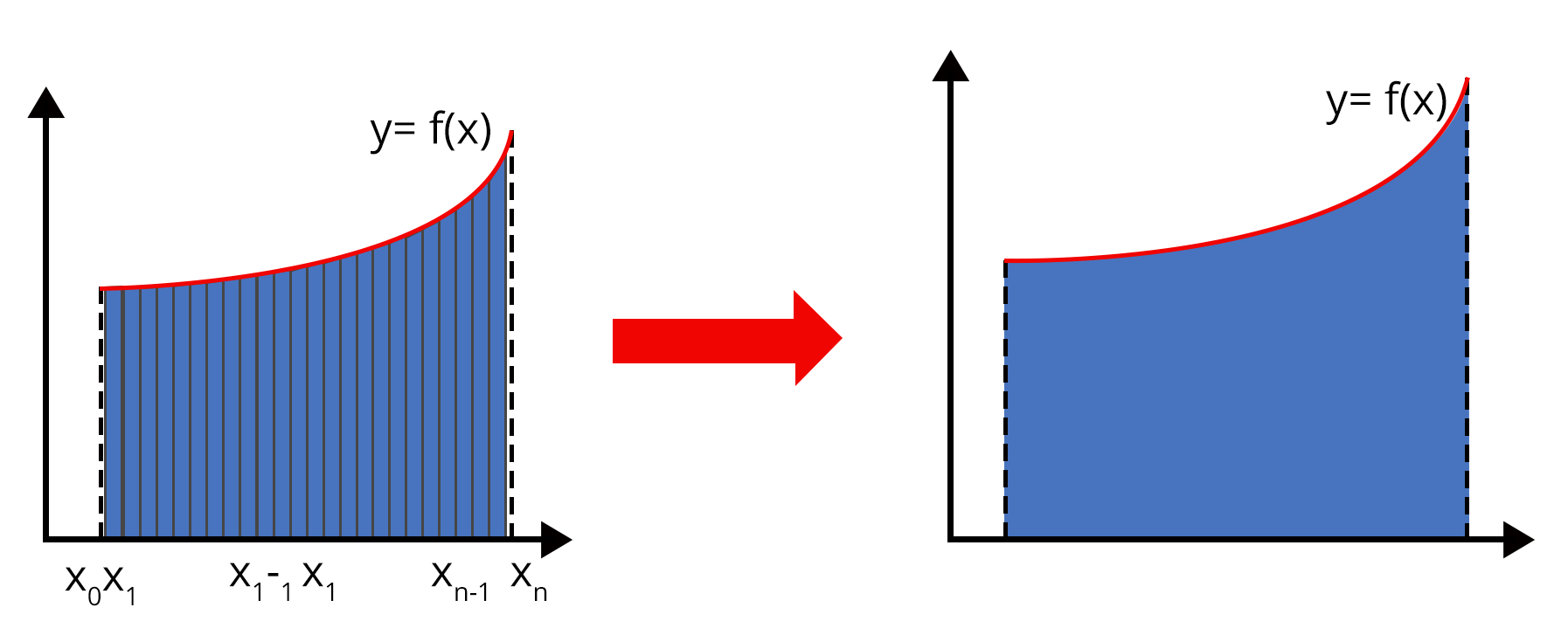Image: Area under the curve Some of integral of basic function is given below$\int dx=x$$\int x^ndx=\dfrac{x^{n+1}}{n+1}+c$$\int \dfrac{1}{x}.dx=\ln x +c$$\int e^xdx=e^x+c$$\int a^xdx=\dfrac{a^x}{\ln a}+c$ 8. Definite integration When an integral is defined between two definite limits a and b, it is said to be a definite integration.$\int_{a}^{b}f(x)dx=[g(x)]^b_a=g(b)-g(a)$

### List of Important Formulae

 Sl. No Name of the Concept Formula 1. Trigonometric formulae $\sin 2A=2\sin A\cos A$$\cos 2A=2\cos^2A-1=1-2\sin^2A=\cos^2A-\sin^2A$$\sin (A+B)+\sin(A-B)=2\sin A\cos B$$\cos(A+B)+\cos(A-B)=2\cos A\cos B 2. Differentiation of trigonometric functions \dfrac{d}{dx}(\tan x)=\sec^2x$$\dfrac{d}{dx}(\cot x)=-\csc^2x$$\dfrac{d}{dx}(\sec x)=\sec x\tan x$$\dfrac{d}{dx}(\csc x)=-\csc x\cot x$ 3. Logarithmic formulae $\ln e=1$$\ln 1=0$$log_{10}10^x=x$$\ln (mn)=\ln m+\ln n$$\ln\left(\dfrac {m}{n}\right)=\ln m-\ln n$ 4. Integration formulae of trigonometric function $\int\cos x.dx=\sin x+c$$\int\sin x.dx=-\cos x+c$$\int\sec^2 x.dx=\tan x+c$$\int\csc^2 x.dx=-\cot x+c$$\int\sec x\tan x.dx=\sec x+c$$\int\csc x\cot x.dx=-\csc x+c 5. Conversion coefficients 1\text{ light year}=9.46\times10^{15}~m$$1A.U=1.496\times10^{11}~m$$1~A=10^{-10}~m$$1\text{ Fermi}=10^{-15}~m$$1eV=1.6\times10^{-19}~J$$1\text{ Horse power}=746~W$

### Solved Examples

1. The position of a particle on x-axis that varies with time is given as x=3t^2+2t+1. Find the velocity of the particle at t=2 s.

Sol:

Given,

The position of the particle ,x=3t2+2t+1

The velocity of particle at t=2 s can be found by differentiating the displacement and substituting the value of t as 2 sec

$v=\dfrac{dx}{dt}$

$v=\dfrac{d}{dt}(3t^2+2t+1)$

$v=3\times2t+2$

$v=6t+2$

Put t=2 to calculate the velocity at that instant.

$v=6t+2$

$v=6\times2+2$

$v=14~m/s$

Therefore, the velocity of the particle at t=2 s is 14 m/s.Key point: Differentiating
displacement with respect to time gives velocity.

2. The variable force acting on a body of mass 1 kg depends on the displacement on x -axis as F=3x2+2. Find the work done by the variable force from x=0 to x= 2m.

Sol:

Given,

The variable force acting on the body, F=3x2+2

We can calculate the work done by the force by integrating force from x=0 m to x= 2m

$W=\int_{0}^{2} F.dx$

$W=\int_{0}^{2} (3x^2+2).dx$

$W=3\dfrac{[x^3]^2_0}{3}+2[x]^2_0$

$W=12~J$

Thus, the work done by the variable force from x=0 to x= 2m is 12J.

Key Point: The work done by a variable force can be obtained by integration of the variable force with respect to displacement.

### Previous Year Questions from NEET Paper

1. A particle moves along a straight line OX. At a time t (in seconds), the distance x (in metres) is given by x=40+12t-t3. How long would the particle travel before coming to rest? (NEET 2006)

a. 24 m

b. 40 m

c. 56 m

d. 16 m

Sol:

The speed of the particle can be obtained by differentiating the distance with respect to time.

$v=\dfrac{dx}{dt}$

$v=\dfrac{d}{dt}(40+12t-t^3)$

$v=0+12-3\times t^2$

$v=-3t^2+12$

When particle comes to rest, the speed of the particle becomes zero

$0=-3t^2+12$

$t^2=4$

$t=2~s$

Now, the distance travelled by the particle is

$x=40+12t-t^3$

$x=40+12\times2-2^3$

$x=56~m$

Therefore, correct answer is option (c)

Trick: Speed of the particle can be obtained by differentiating the expression for distance with respect to time.

2. The displacement of a particle moving in a straight line is given by s=2t2+2t+4, where s is in metres and t is in seconds. The acceleration of the particle is (NEET 2001)

1. 2 m/s2

2. 4 m/s2

3. 6 m/s2

4. 8 m/s2

Sol:

The speed of the particle can be obtained by

$v=\dfrac{dx}{dt}$

$v=\dfrac{d}{dt}(2t^2+2t+4)$

$v=4t+2$

The acceleration of the particle is calculated as follows

$a=\dfrac{dv}{dt}$

$a=\dfrac{d}{dt}(4t+2)$

$v=4~m/s^2$

Therefore, correct answer is option (b)

Trick: When displacement of any object is given second-order differentiation will

be the acceleration of the object.

### Practice Questions

1. Find the work done to elongate a spring having a spring constant of 2000N/m from an extension of 1 cm to 2 cm.

Ans: 0.3 J

2. If v=x2-5x+4, find the acceleration of the particle when the velocity of the particle is zero.

Ans: 0 m/s2

### Conclusion

In this article, we have provided important information under several mathematical tool names necessary for solving numerical problems in Physics. Students should have a better understanding of the mathematical operations so that students can score good marks in Physics for the NEET exam.

See More## NEET Important Dates

View All Dates
NEET 2022 exam date and revised schedule have been announced by the NTA. NEET 2022 will now be conducted on 17-July-2022, and the exam registration closes on 20-May-2022. You can check the complete schedule on our site. Furthermore, you can check NEET 2022 dates for application, admit card, exam, answer key, result, counselling, etc along with other relevant information.
See More
View All Dates## NEET Information

Application Form
Eligibility Criteria
Reservation Policy
NTA has announced the NEET 2022 application form release date on the official website https://neet.nta.nic.in/. NEET 2022 Application Form is available on the official website for online registration. Besides NEET 2022 application form release date, learn about the application process, steps to fill the form, how to submit, exam date sheet etc online. Check our website for more details.## NEET 2022 Study Material

View NEET Syllabus in Detail
View NEET Syllabus in Detail## NEET 2022 Study Material

View all study material for NEET
All
Physics
Chemistry
Biology
See All## NEET Question Papers## NEET 2022 Book Solutions and PDF Download

View all NEET Important Books
Biology
NCERT Book for Class 12 Biology
Physics
NCERT Book for Class 12 Physics
Chemistry
NCERT Book for Class 12 Chemistry
Physics
H. C. Verma Solutions
See All## NEET Mock Tests

View all mock tests
"NEET 2022 free online mock test series for exam preparation are available on the Vedantu website for free download. Practising these mock test papers of Physics, Chemistry and Biology prepared by expert teachers at Vedantu will help you to boost your confidence to face the NEET 2022 examination without any worries. The NEET test series for Physics, Chemistry and Biology that is based on the latest syllabus of NEET and also the Previous Year Question Papers."
See More## NEET 2022 Cut Off

NEET Cut Off
NTA is responsible for the release of the NEET 2022 cut off score. The qualifying percentile score might remain the same for different categories. According to the latest trends, the expected cut off mark for NEET 2022 is 50% for general category candidates, 45% for physically challenged candidates, and 40% for candidates from reserved categories. For the general category, NEET qualifying marks for 2021 ranged from 720-138 general category, while for OBC/SC/ST categories, they ranged from 137-108 for OBC, 137-108 for SC and 137-108 for ST category.
See More## NEET 2022 Results

The NEET 2022 result is published by NTA on https://neet.nta.nic.in/ in the form of a scorecard on 7-Sep-2022. The scorecard will include the roll number, application number, candidate's personal details, and the percentile, marks, and rank of the candidate. Only those candidates who achieve the NEET cut-off will be considered qualified for the exam.
See More
Rank List
Counselling
Cutoff
NEET 2022 state rank lists will be released by the state counselling committees for admissions to the 85% state quota and to all seats in private medical and dental colleges. NEET 2022 state rank lists are based on the marks obtained in entrance exams. Candidates can check the NEET 2022 state rank list on the official website or on our site.## NEET Top Colleges

View all NEET 2022 Top Colleges
Want to know which Engineering colleges in India accept the NEET 2022 scores for admission to Engineering? Find the list of Engineering colleges accepting NEET scores in India, compiled by Vedantu. There are 1622 Colleges that are accepting NEET. Also find more details on Fees, Ranking, Admission, and Placement.
See More## FAQs on NEET Important Topic - Physics Mathematical Tools

FAQ

1. What are the main mathematical tools required in Physics for the NEET exam?

The main mathematical tools required for Physics in the NEET exam are differentiation and its applications, indefinite and definite integrations, trigonometric functions and their identities, measurement of positive and negative angles etc. You can download the mathematics tool pdf from Vedantu for easy reference.

2. Is Calculus required for NEET?

Some of the problems in Physics require a basic understanding of Calculus to solve problems in chapters like electrostatics, the system of particles, magnetic effects of current, etc. Even though students don't have to memorise all the Calculus formulas, they must know how to apply Calculus in a given situation to find the solution to the problems.

3. How to score good marks in Physics for the NEET exam?

First of all, understand all the concepts in depth and practise all the numerical problems in the NCERT textbook as well as other good study materials. Then, work out all the questions in the previous year's question papers. These will ensure that you will get good marks in physics for the NEET exam.## Notice board

News
BlogTrending pages